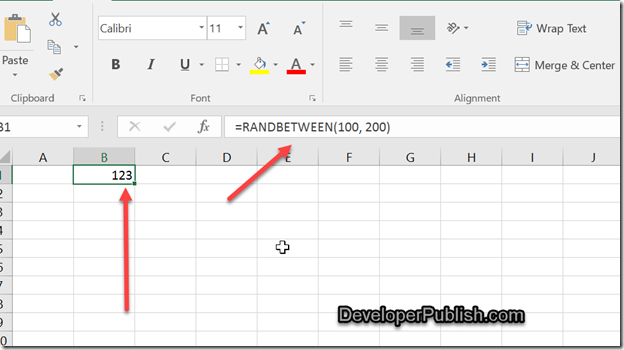# How to insert Random Number between 2 numbers in Microsoft Excel ?

Do you want to insert a random number between 2 numbers in a excel cell? For example , assume that you want to display a random number in the cell B1 which is in between the values 100 and 200 , you can use the excel function RANDBETWEEN.

# How to insert Random Number between 2 numbers in Microsoft Excel ?

1. Select a cell where you want to display the random number and enter the following formula and press the enter key.

=RANDBETWEEN(100, 200)

The first parameter is the lowest limit and the second parameter is the maximum limit within which you want to generate the random number.

You should immediately see the random number displayed in the cell.